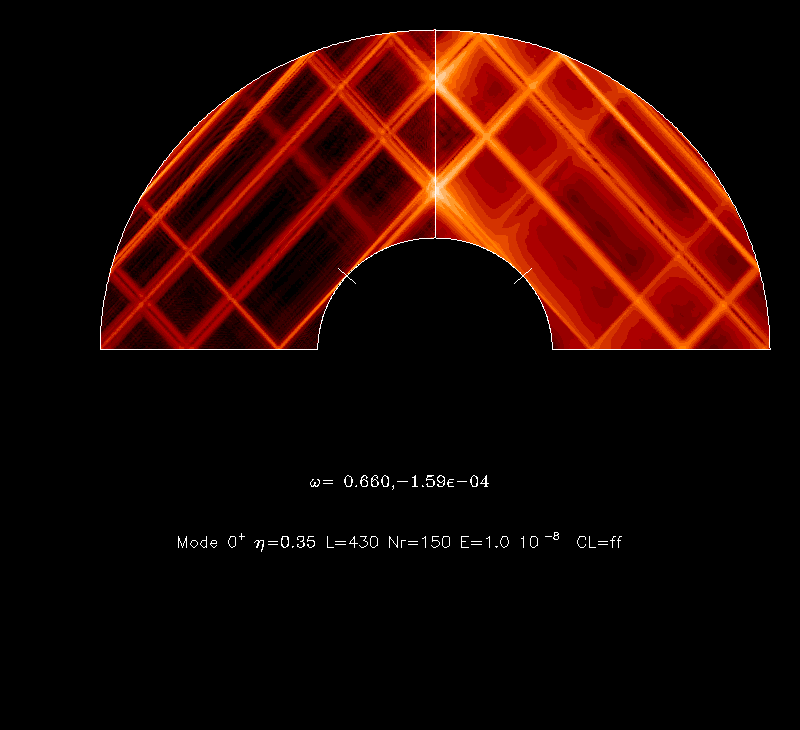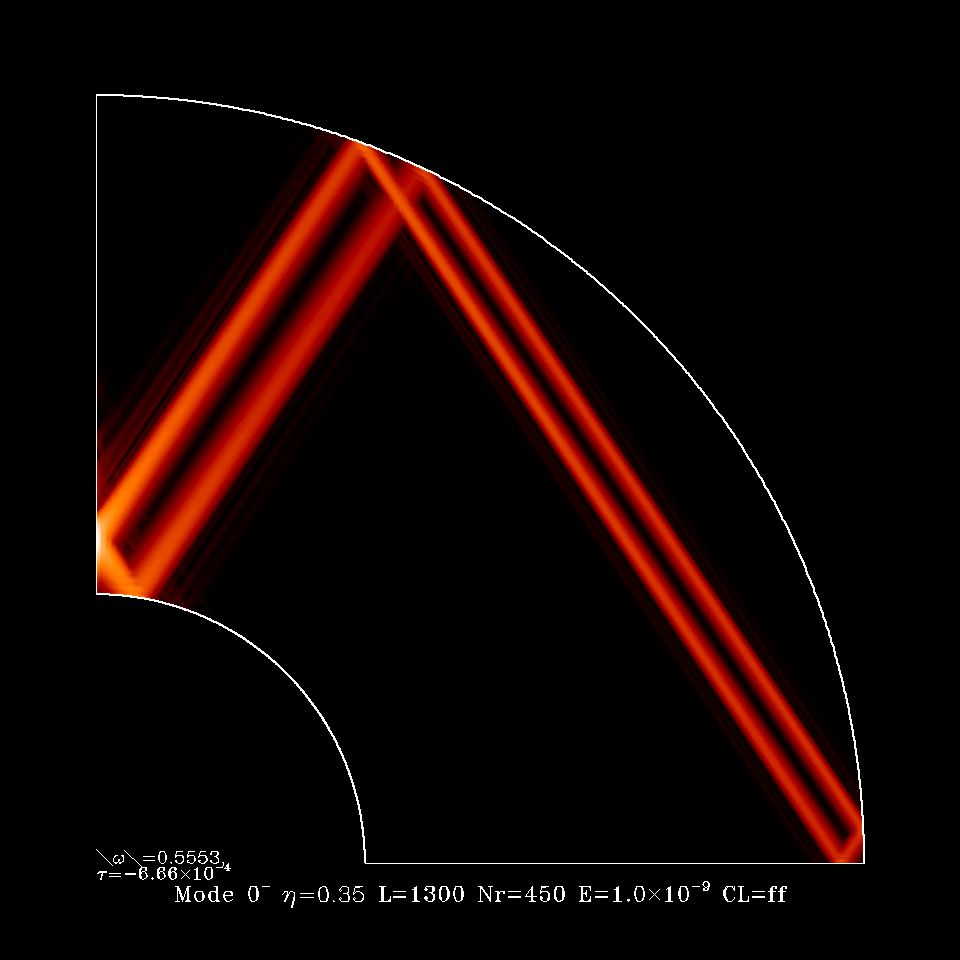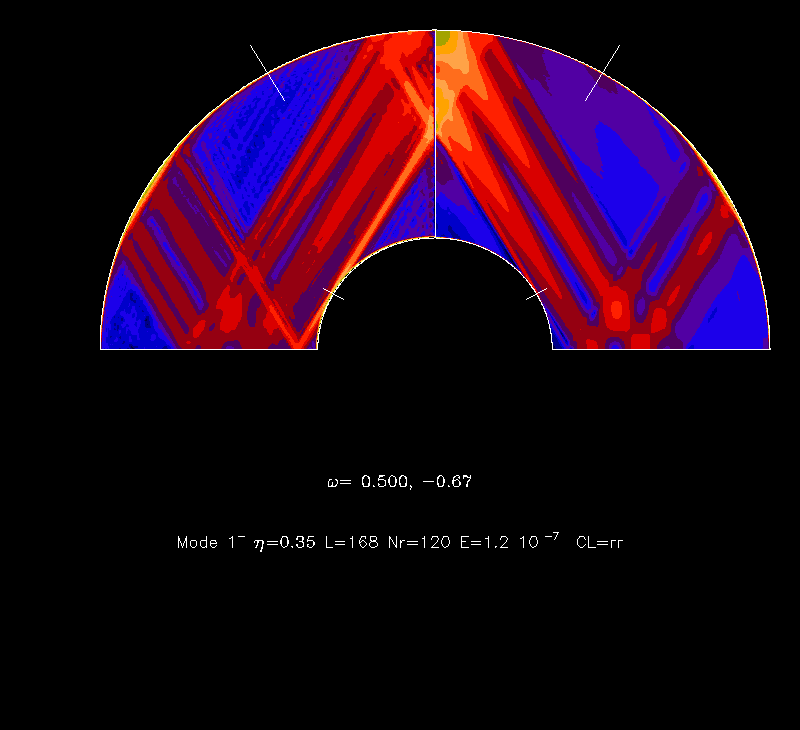Rotating Fluids: the theory

In astrophysical and geophysical fluids rotation is often playing an important part. In these common cases, the Coriolis force is the dominant force. Fluid motions under this force show a tendancy of being invariant with respect to the coordinate along the axis of rotation. This is a consequence of the so-called Taylor-Proudman theorem which applies to quasi-steady flows.
When the flows are not steady, but the Coriolis force is still dominating all other effects (nonlinearities or viscosity), then we are facing wave motions, namely inertial waves, for which the Coriolis force is the restoring force. Such waves have very strange properties: the phase and the energy propagate in perpendicular directions! In addition, when the fluid is contained in some finite volume, the mode of oscillation of the fluid obey the Poincaré equation which is a hyperbolic equation. This is strange because the Poincaré equation governs the pressure perturbations which meet boundary conditions. A hyperbolic equation plus boundary conditions make an ill-posed boundary value problem as mathematicians say. However, such ill-posed problems are quite difficult and, for instance, the solutions of the Poincaré equation, when the fluid is in a spherical shell, have been uncovered only recently (Rieutord and Valdettaro 1997, Rieutord et al. 2001), while they have been known for more than a century for the full sphere (Bryan 1888).
The difficulty of this problem comes from its 'ill-posedness' and the non-separability of the variables for containers like the spherical shell: this yields many singularities; in fact all solutions but a few ones are singular. These singularities generate shear layers when the viscosity of the fluid is taken into account. These singularities are the 'rays' which show up in a meridional plane of a spherical shell when the kinetic energy density is plotted. This is what is shown on the figures below